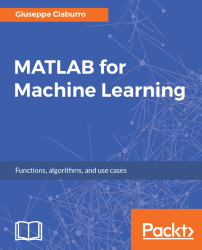•#### MATLAB for Machine Learning#### Overview of this book

MATLAB is the language of choice for many researchers and mathematics experts for machine learning. This book will help you build a foundation in machine learning using MATLAB for beginners. You’ll start by getting your system ready with t he MATLAB environment for machine learning and you’ll see how to easily interact with the Matlab workspace. We’ll then move on to data cleansing, mining and analyzing various data types in machine learning and you’ll see how to display data values on a plot. Next, you’ll get to know about the different types of regression techniques and how to apply them to your data using the MATLAB functions. You’ll understand the basic concepts of neural networks and perform data fitting, pattern recognition, and clustering analysis. Finally, you’ll explore feature selection and extraction techniques for dimensionality reduction for performance improvement. At the end of the book, you will learn to put it all together into real-world cases covering major machine learning algorithms and be comfortable in performing machine learning with MATLAB.
Title PageCreditsForewordwww.PacktPub.comCustomer FeedbackPrefaceFree Chapter
Getting Started with MATLAB Machine LearningImporting and Organizing Data in MATLABFrom Data to Knowledge DiscoveryFinding Relationships between Variables - Regression TechniquesPattern Recognition through Classification AlgorithmsIdentifying Groups of Data Using Clustering MethodsSimulation of Human Thinking - Artificial Neural NetworksImproving the Performance of the Machine Learning Model - Dimensionality ReductionMachine Learning in Practice## Summary

In this chapter, we learned how to perform an accurate classification in a MATLAB environment. First, we explored decision trees methods; we learned concepts like nodes, branches, and leaf nodes. We saw how to classify objects into a finite number of classes by repeatedly dividing the records into homogeneous subsets with respect to the target attribute. Then, we looked at how to predict a response with decision trees.

Next, we discovered the probabilistic classification algorithm that determines the probability that an element belongs to a particular class. We learned the basic concepts of probability theory: classical probability definition, dependent and independent events, joint probability and conditional probability, which is the basis of these methods. Then, we understood how to classify with the Naive Bayes algorithm.

We explored discriminant analysis methodologies; several examples were analyzed to compare the relative results. We learned how to create models to minimize the...